# LED tutorial

As discussed in #1217, it might be nice to have a tutorial with e.g. a 2d LED-like structure with a thin (1d) light-emitting layer, showing several ways to solve it:

1. Brute force: just a layer of random dipole emitters. By linearity, we can make them white noise and then scale by a desired spectrum later. (Similar to Alejandro's first near-field heat-transfer paper.) You'll need lots of simulations (and/or a long time) to get the spectrum to good accuracy (low variance).

2. Better: since the dipoles are uncorrelated, we can just do a sequence of deterministic calculations, with one dipole at a time, for dipoles at different points — uncorrelated dipoles at different points just add their powers. So, you compute the powers and (suitably normalized, similar to how LDOS is normalized) add them up, scaling by the desired spectrum.

We could mention other techniques:

1. Even better: instead of summing dipoles, we could use a different set of basis functions, e.g. a cosine series. See Alex McCauley's Casimir FDTD paper.

2. Even better, for the case of volumetric emitters: use Alejandro and Homer's tricks to transform a volume of emitters into equivalent surface emitters. Or just use SCUFF.

For example, you could look at a structure like this one: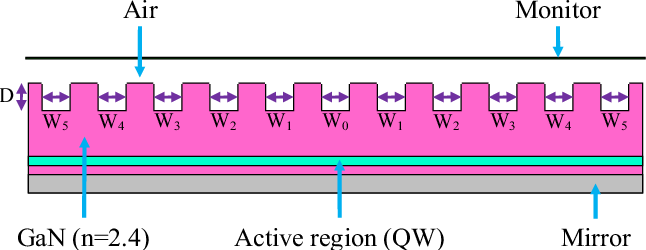but with all widths W equal so that it's periodic. Compute the emission in the normal direction by treating the emitting layer as infinitesimally thin. This allows you to model a single unit cell with periodic (k=0) boundary conditions. (Emission into other directions can be computed by changing k.)

• 点赞
• 写回答
• 关注问题
• 收藏
• 复制链接分享
• 邀请回答

#### 26条回答

• Yes; changing the origin of the source solved it.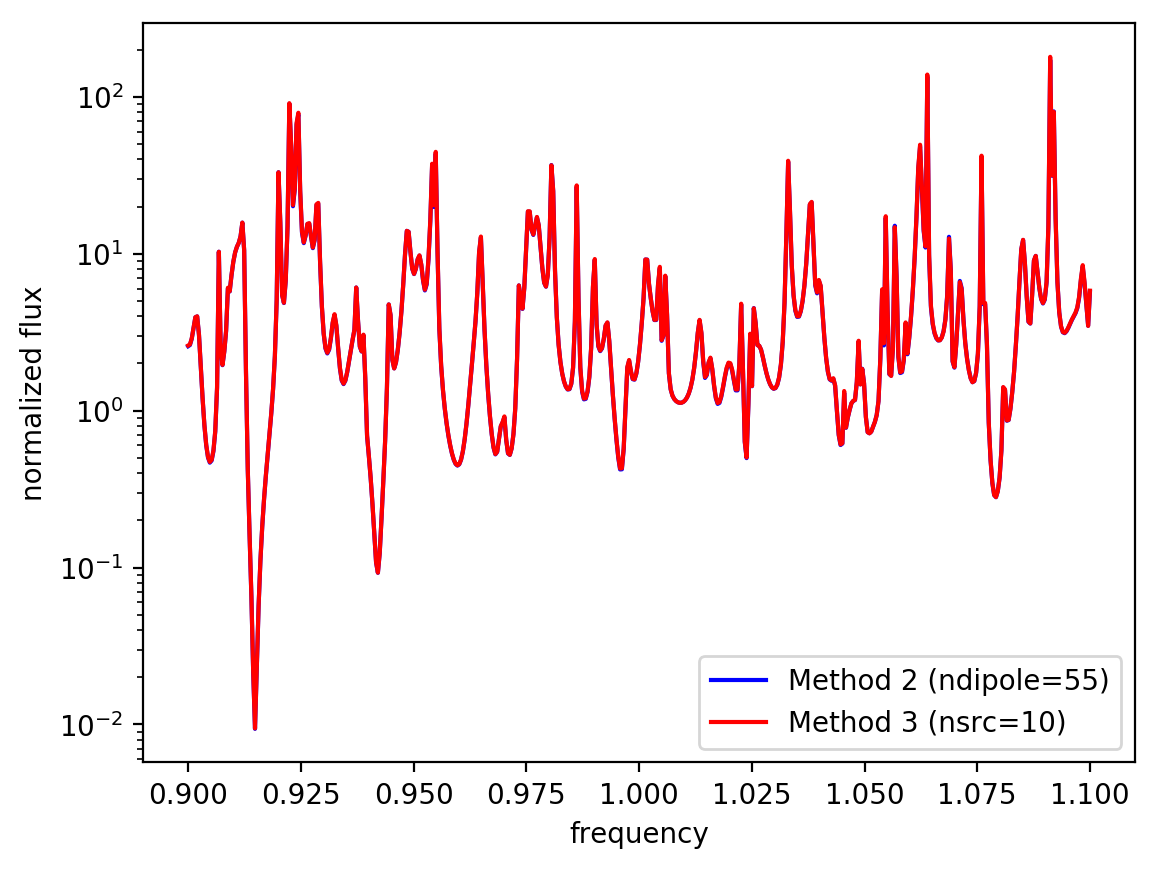点赞 评论 复制链接分享
• Initial results comparing Method 2 and 3 for the 2d LED structure from above. Method 2 involves 55 single dipole calculations (`resolution*L` where `resolution=50`, `L=1.1`) which takes about 3.5 hours (for the flat/textured surface). Method 3 involves N=10 calculations with line sources having an amplitude function based on a cosine series (using the formula for fn(x) in the Casimir tutorial) which takes about 0.6 hours. The source time profile is identical in both cases (Gaussian pulse) and the runtime is 2/frequency resolution (same as before).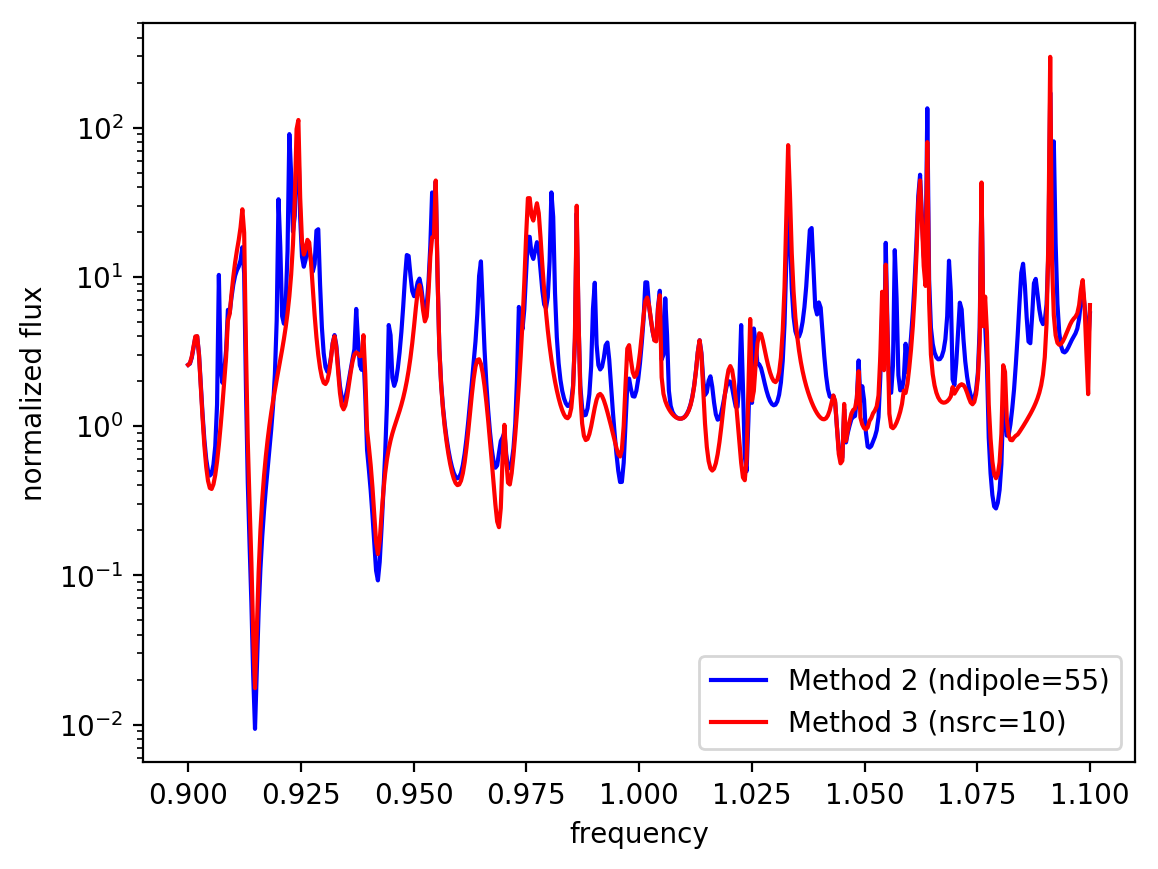The agreement between Method 2 and 3 is not as good as it was between Method 1 and 2 shown previously. The Method 3 results are converged for N=10 as shown in the plot below for N=5, 10, and 20.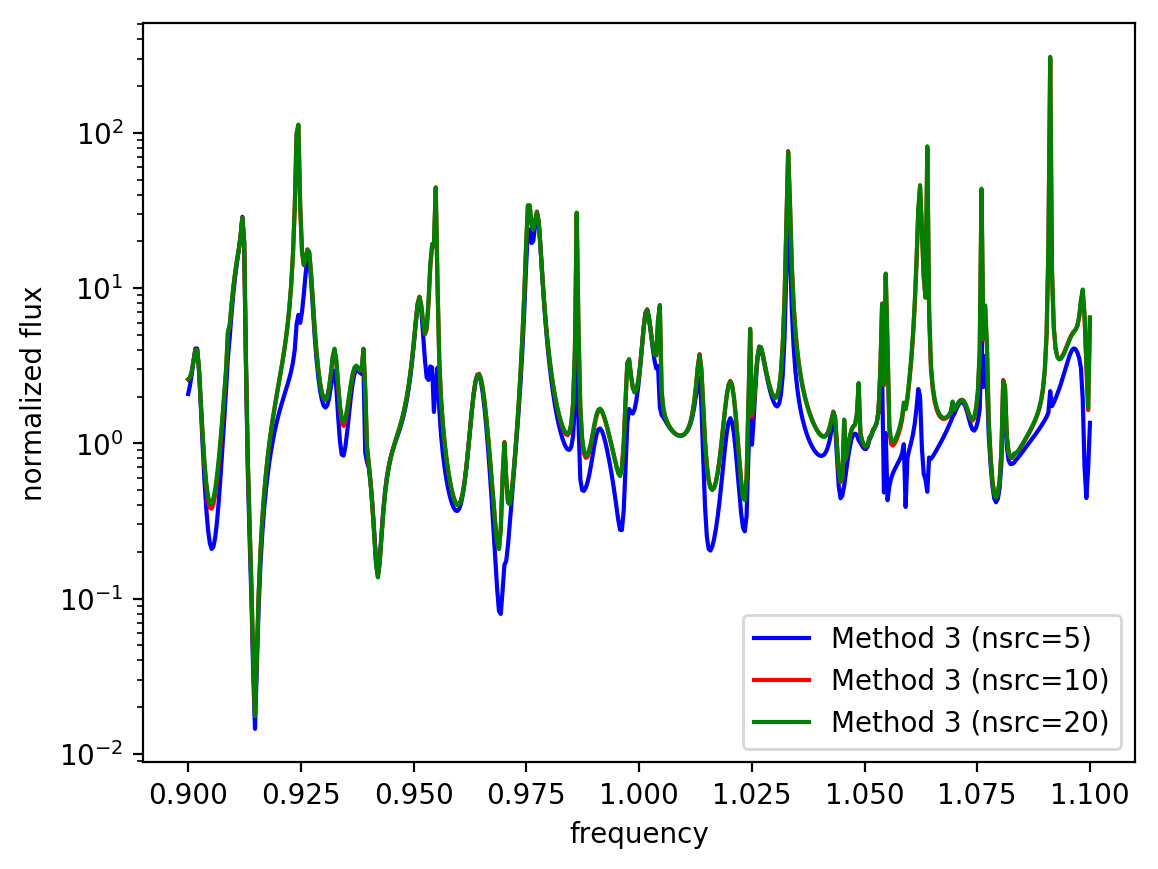It is possible (but unlikely) that results for Method 2 are still not converged with respect to the runtime of 2/frequency resolution. Should we try increasing the resolution?

点赞 评论 复制链接分享
• The source used for Method 3:

``````py
def src_amp_func(n):
def _src_amp_func(p):
if n == 0:
return 1/np.sqrt(sx)
else:
return np.sqrt(2/sx) * np.cos(n*np.pi*p.x/sx)
return _src_amp_func

def compute_flux(n):
sources = [mp.Source(mp.GaussianSource(fcen,fwidth=df),
component=mp.Ez,
center=mp.Vector3(0,-0.5*sy+dAg+0.5*dsub),
size=mp.Vector3(sx,0),
amp_func=src_amp_func(n))]

sim = mp.Simulation(cell_size=cell_size,
resolution=resolution,
k_point=mp.Vector3(),
boundary_layers=pml_layers,
geometry=geometry,
sources=sources)

sim.run(until_after_sources=run_time)
flux = mp.get_fluxes(flux_mon)
freqs = mp.get_flux_freqs(flux_mon)

return freqs, flux

``````
点赞 评论 复制链接分享
• I think you have an origin problem. In the Casimir case and in the Fourier cosine series as it is conventionally written, i.e. cos(πx/L) is define so that x=[0,L] … i.e. x=0 corresponds to the edge of the source, not the center.

So, you just need to change `p.x` to `p.x + sx/2` in your amplitude function.

点赞 评论 复制链接分享
• Increasing the runtime for method 2 does indeed improve the resolution of the sharp peaks. The agreement with method 1 is now much better.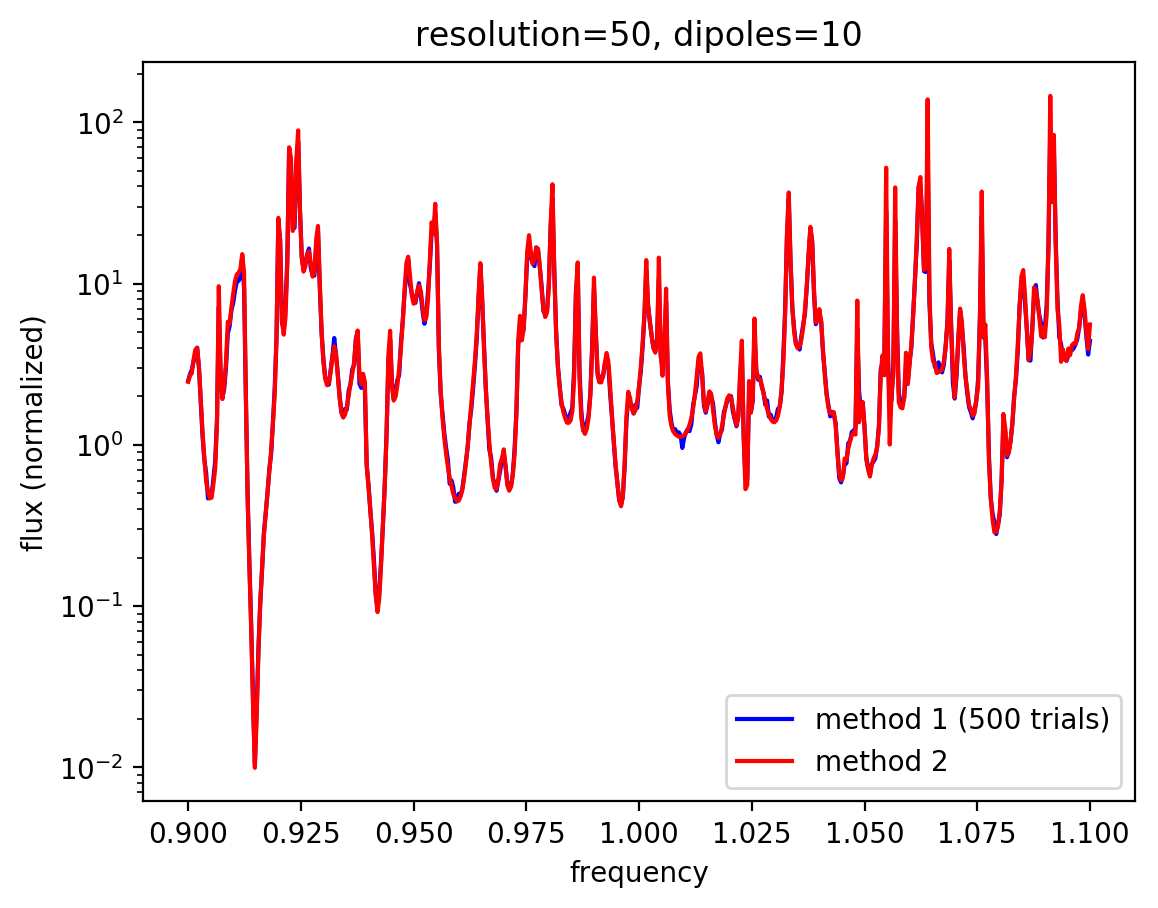点赞 评论 复制链接分享
• It would be nice to add a tutorial comparing method 2 to method 3.

That is, you have a line of length L of uncorrelated emitters.

1. In method 2, you would use `L*resolution` point sources, one per pixel.

2. In method 3, you would use a sequence of line sources with cosine amplitude functions, exactly as described in the "Sources" section of the Casimir tutorial, for n=0, 1, 2,…,N

In both cases, you add up the powers from each simulation.

In method 3, keep doubling N until the answer stops changing to a desired tolerance, e.g. < 1%. You should find that N is essentially independent of resolution, in contrast to method 2 where the number of sources increases with resolution.

To start with, you could normalize against a flat surface as before. However, if you normalize the cosine terms correctly (I think the same as in Alex McCauley's notes), I think you should find that you obtain the same absolute sum even without normalizing. (Well, there is also probably a factor of `resolution` that you have to multiply by in the point-source sum.)

点赞 评论 复制链接分享
• (If the line sources are periodic, I think it should be even more efficient to use a sine+cosine Fourier-series basis rather than a cosine-only basis. But we can do cosines to start with since they are more generic.)

点赞 评论 复制链接分享
• Based on the results shown below, it seems we can demonstrate fairly good agreement between method 1 and 2. It's likely that the sharp peaks/dips for method 1 are simply an artifact of the number of random trials (500) and the agreement can be improved further by using an even larger number of trials. Because the flux spectra for the textured surface is normalized relative to the flat surface, there is no need for additional scaling in post processing.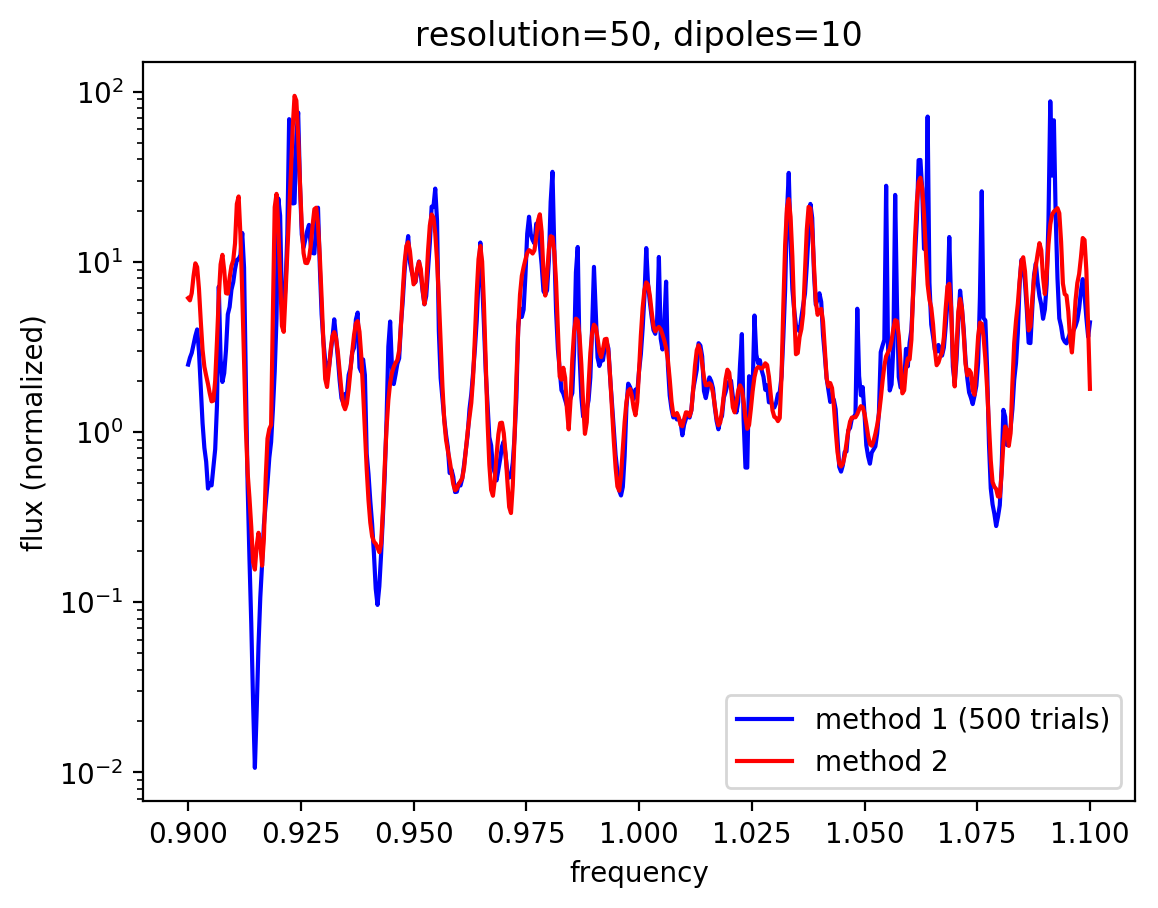For now, the results we have compiled thus far should be sufficient for the tutorial. The next step is to add the results for methods 3 and 4.

点赞 评论 复制链接分享
• Is it converged with runtime for method 2? You might need a longer run to resolve those sharp peaks.

点赞 评论 复制链接分享
• (If we eventually want to show the cosine-series method, there should be one dipole per pixel along the source line, so that the number of dipoles increases with resolution.)

点赞 评论 复制链接分享
• The following are three sets of results (10, 50, and 320 trials) for the normalized flux (textured/flat) on a semilogy plot. The fuzziness is reduced with increasing number of trials but the changes are not so significant.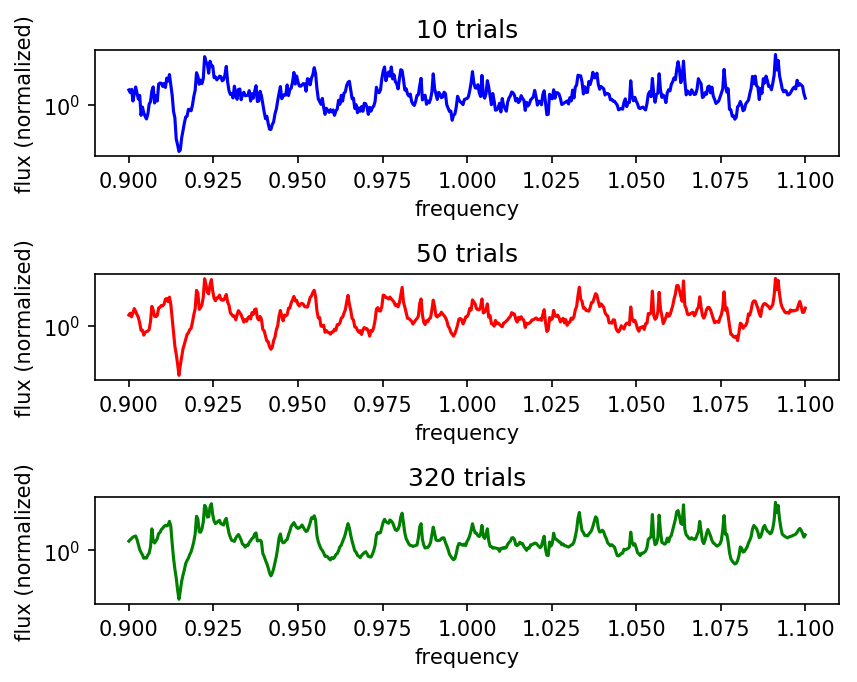点赞 评论 复制链接分享
• Using normalization approach 1, these are the results for the flat and textured emitters averaged over 500 random trials separately (i.e., the denominator and numerator).

flat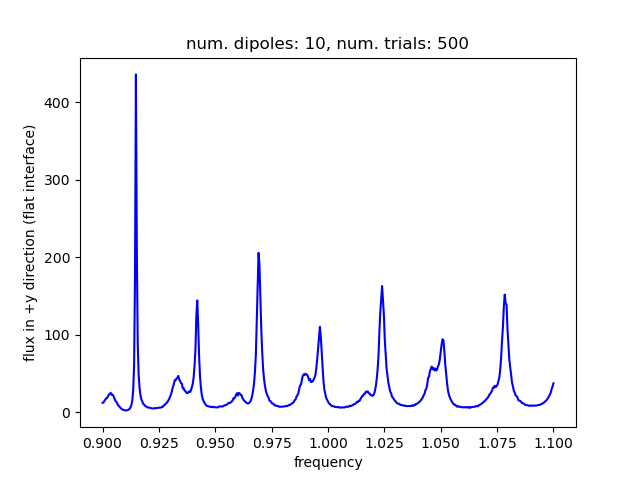textured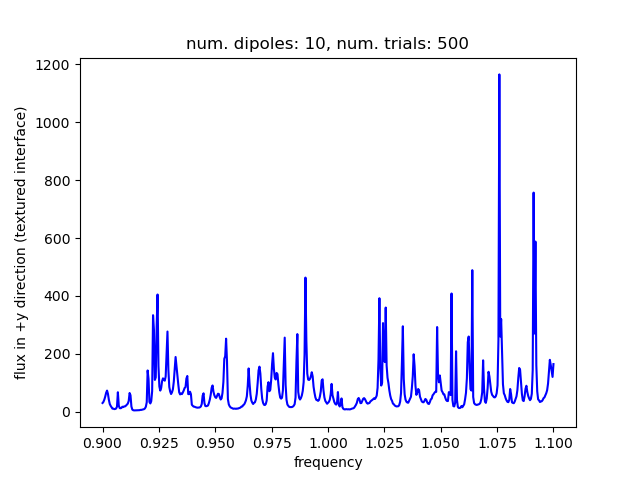点赞 评论 复制链接分享
• What are you normalizing against? A homogeneous medium?

点赞 评论 复制链接分享
• If you look carefully, you can see some bumpiness. It might be instructive to show the spectra for 100 trials superimposed underneath the 500-trial curve.

点赞 评论 复制链接分享
• Switching to a `CustomSrc` and running for 2/(frequency resolution) as well as increasing the number of trials to 500 (which required 3 days to run) makes the spectral peaks more pronounced with practically no noise or "fuzziness":

``````py
sources = []
for n in range(ndipole):
sources.append(mp.Source(mp.CustomSource(src_func=lambda t: np.random.randn()),
component=mp.Ez,
center=mp.Vector3(sx*(-0.5+n/ndipole),-0.5*sy+dAg+0.5*dglass)))
...
...
...
run_time = 2*nfreq/df
sim.run(until=run_time)
``````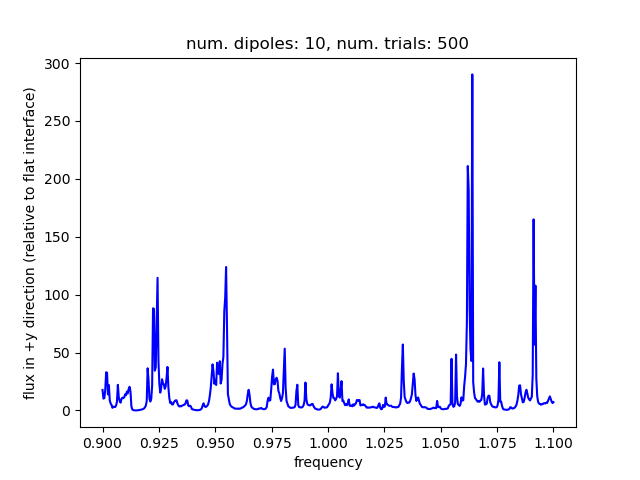点赞 评论 复制链接分享
• You definitely want normalization 1, not normalization 2. You want to divide the ensemble-averaged fields. (And no need for the random numbers to be the same in F and F0.)

点赞 评论 复制链接分享
• After increasing the number of frequencies by nearly a factor of 10 (from 51 to 501), two comments on the results shown below:

1. the noisy spectrum of method 1 is not apparent and increasing the runtime did not the change results significantly
2. the spectra from the two methods still differ by an overall scale factor even though both sets of results are normalized relative to the flat surface termination.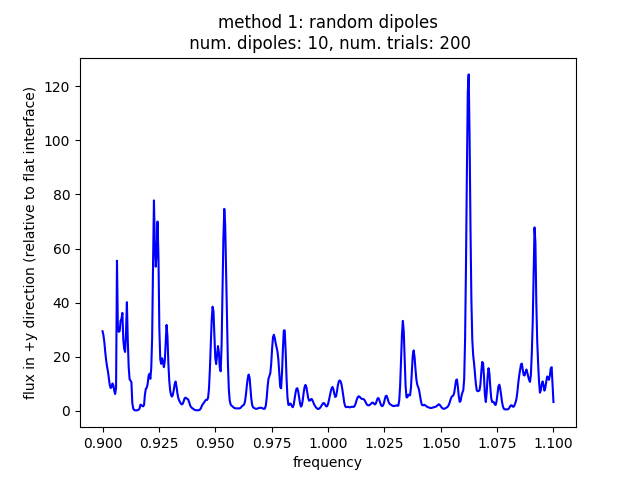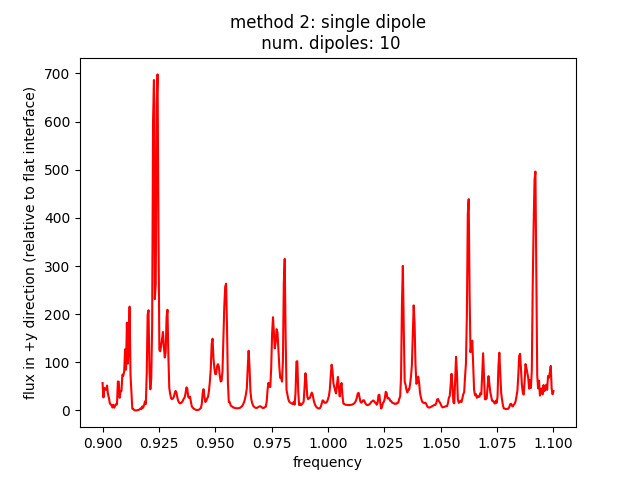Perhaps we need to go to an even finer frequency discretization and look at a narrower bandwidth in order to resolve the tiny fluctuations?

点赞 评论 复制链接分享
• Probably you need a much longer runtime (~ 1/frequency resolution) to see the noise in the monte-carlo spectrum.

点赞 评论 复制链接分享
• I think there was a misunderstanding, in method 1, you need white-noise sources. Every timestep for every dipole should be an independent random number.

点赞 评论 复制链接分享
• That is, something like `CustomSrc(src_func=lambda t: np.random.randn())` for each dipole. (Real fields is fine.)

点赞 评论 复制链接分享
• Two questions regarding the implementation strategy for method 1 (random dipoles).

Q1 There are two different ways to perform the normalization given the flux spectrum for the flat surface (F0) and corrugated surface (F) over n trials: 1. (Σₙ F) / (Σₙ F0) 2. Σₙ (F / F0)

These two approaches do not appear to be equivalent. Thus far, I've been using (2). Is this correct?

(The same normalization approach is used for method 2.)

Q2 For a given trial, are we supposed to use the same random source to compute F and F0? If so, this would require storing the random numbers from the first run involving F0 and then reusing them to compute F. Thus far, F and F0 are computed using a unique (randomly generated) `CustomSource`.

点赞 评论 复制链接分享
• A comparison of the nondimensionalized spectra for (1) and (2) shows that, aside from a couple of extra peaks in (1) even when using 200 random trials, they are similar and differ by an overall constant factor. The number of dipoles as well their locations is the same in both methods.

To obtain exact agreement, perhaps (1) requires an even larger number of random trials (i.e. >> 200 which already takes a few hours) and/or a different uncorrelated random number generator than `random.random()`?

``````
import meep as mp
from meep.materials import Ag

import numpy as np
import random

import matplotlib
matplotlib.use('agg')
import matplotlib.pyplot as plt

import argparse
parser = argparse.ArgumentParser()
parser.add_argument('-nd', type=int, default=10, help='number of dipoles')
args = parser.parse_args()

resolution = args.res

dpml = 1.0
dair = 1.0
hrod = 0.7
wrod = 0.5
dglass = 5.0
dAg = 0.5

sx = 1.1
sy = dpml+dair+hrod+dglass+dAg

cell_size = mp.Vector3(sx,sy)

pml_layers = [mp.PML(direction=mp.Y,
thickness=dpml,
side=mp.High)]

fcen = 1.0
df = 0.2
nfreq = 51

geometry = [mp.Block(material=mp.Medium(index=3.45),
center=mp.Vector3(0,0.5*sy-dpml-dair-0.5*hrod),
size=mp.Vector3(wrod,hrod,mp.inf)),
mp.Block(material=mp.Medium(index=3.45),
center=mp.Vector3(0,0.5*sy-dpml-dair-hrod-0.5*dglass),
size=mp.Vector3(mp.inf,dglass,mp.inf)),
mp.Block(material=Ag,
center=mp.Vector3(0,-0.5*sy+0.5*dAg),
size=mp.Vector3(mp.inf,dAg,mp.inf))]

geometry_ref = [mp.Block(material=mp.Medium(index=3.45),
center=mp.Vector3(0,0.5*sy-dpml-dair-hrod-0.5*dglass),
size=mp.Vector3(mp.inf,dglass,mp.inf)),
mp.Block(material=Ag,
center=mp.Vector3(0,-0.5*sy+0.5*dAg),
size=mp.Vector3(mp.inf,dAg,mp.inf))]

def compute_flux(n,ndipole):
sources = [mp.Source(mp.GaussianSource(fcen,fwidth=df),
component=mp.Ez,
center=mp.Vector3(sx*(-0.5+n/ndipole),-0.5*sy+dAg+0.5*dglass))]

sim = mp.Simulation(cell_size=cell_size,
resolution=resolution,
k_point=mp.Vector3(),
boundary_layers=pml_layers,
geometry=geometry_ref,
sources=sources)

sim.run(until_after_sources=500)
flux_ref = mp.get_fluxes(flux_mon)

sim.reset_meep()

sim = mp.Simulation(cell_size=cell_size,
resolution=resolution,
k_point=mp.Vector3(),
boundary_layers=pml_layers,
geometry=geometry,
sources=sources)

sim.run(until_after_sources=500)
flux = mp.get_fluxes(flux_mon)
freqs = mp.get_flux_freqs(flux_mon)

flux_norm = [f/f_ref for f_ref,f in zip(flux_ref,flux)]
sim.reset_meep()

return freqs, flux_norm

ndipole = args.nd
fluxes = np.zeros((nfreq,ndipole))

for n in range(ndipole):
freqs, fluxes[:,n] = compute_flux(n,ndipole)

flux_sum = np.sum(fluxes,axis=1)

if mp.am_master():
plt.figure()
plt.plot(freqs,flux_sum,'bo-')
plt.xlabel('frequency')
plt.ylabel('flux in +y direction (relative to flat interface)')
plt.title('resolution: {}, num. dipoles: {}'.format(resolution,ndipole))
plt.savefig('led_method2_res{}_ndipole{}.png'.format(resolution,ndipole))

for n in range(nfreq):
print("flux:, {}, {}".format(freqs[n],flux_sum[n]))
``````

method 1: random dipoles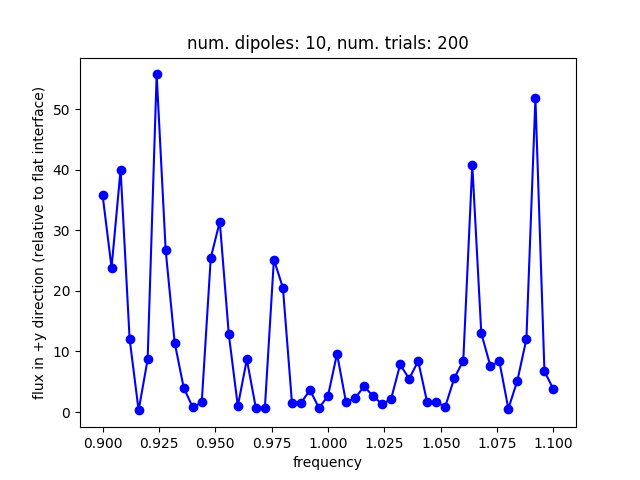method 2: individual dipoles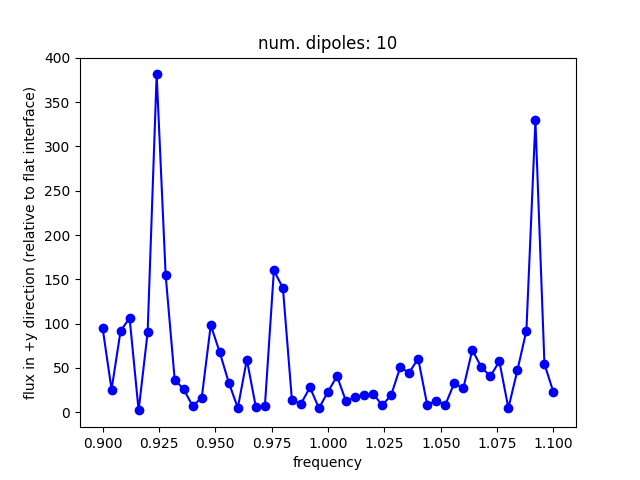点赞 评论 复制链接分享
• Probably you should use a finer frequency discretization so that it is clear that in the individual-dipole case you have a smooth spectrum whereas in the stochastic case you have a noisy spectrum (the real spectrum multiplied by white noise).

点赞 评论 复制链接分享
• (It's only when you average over a large enough ensemble that the noise variance will become small. Note you'll need to run for a long enough time for the DFT spectrum to resolve these oscillations.)

点赞 评论 复制链接分享
• Some initial results for the brute-force approach described in (1). The calculation involves computing the upward flux in a 2d unit cell for a structure similar to that shown above. The setup is shown in the schematic below. The source consists of 10 individual dipole emitters with random complex amplitude (unit magnitude) placed along a line in the emitting layer (red dots). There is a Ag back reflector below the emitter layer so that all the flux is reflected upwards (+y). The flux spectrum is averaged over `N` separate runs and seems to be mostly converged for `N` ~ 40. The flux spectrum for `N` of 20 and 40 are shown below. (A suitable metric for quantifying the convergence could be the L2 norm of the frequency spectra using the reference spectra at `N` of 200.)

The next step is to reproduce these results using the deterministic approach outlined in (2).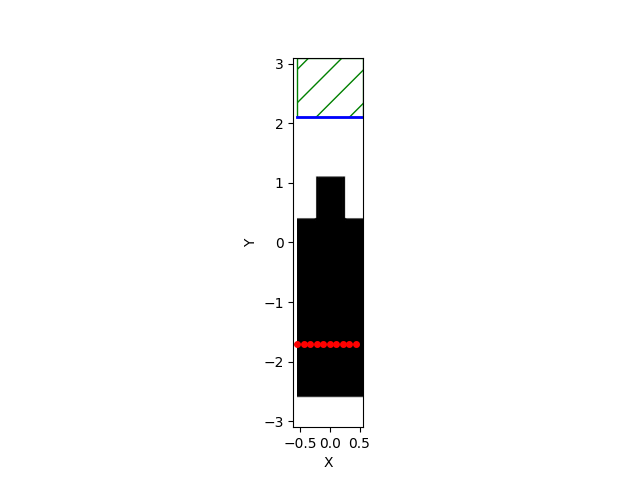``````py
import meep as mp
from meep.materials import Ag

import numpy as np
import random

import matplotlib
matplotlib.use('agg')
import matplotlib.pyplot as plt

import argparse
parser = argparse.ArgumentParser()
parser.add_argument('-nr', type=int, default=20, help='number of flux calculations')
args = parser.parse_args()

resolution = 50

dpml = 1.0
dair = 1.0
hrod = 0.7
wrod = 0.5
dglass = 3.0
dAg = 0.5

sx = 1.1
sy = dpml+dair+hrod+dglass+dAg

cell_size = mp.Vector3(sx,sy)

pml_layers = [mp.PML(direction=mp.Y,
thickness=dpml,
side=mp.High)]

fcen = 1.0
df = 0.1
nfreq = 51
ndipole = 10

geometry = [mp.Block(material=mp.Medium(index=3.45),
center=mp.Vector3(0,0.5*sy-dpml-dair-0.5*hrod),
size=mp.Vector3(wrod,hrod,mp.inf)),
mp.Block(material=mp.Medium(index=3.45),
center=mp.Vector3(0,0.5*sy-dpml-dair-hrod-0.5*dglass),
size=mp.Vector3(mp.inf,dglass,mp.inf)),
mp.Block(material=Ag,
center=mp.Vector3(0,-0.5*sy+0.5*dAg),
size=mp.Vector3(mp.inf,dAg,mp.inf))]

def compute_flux():
sources = []
for n in range(ndipole):
sources.append(mp.Source(mp.GaussianSource(fcen,fwidth=df),
component=mp.Ez,
center=mp.Vector3(sx*(-0.5+n/ndipole),-0.5*sy+dAg+0.3*dglass),
amplitude=np.exp(1j*2*np.pi*random.random())))

sim = mp.Simulation(cell_size=cell_size,
force_complex_fields=True,
resolution=resolution,
k_point=mp.Vector3(),
boundary_layers=pml_layers,
geometry=geometry,
sources=sources)

sim.run(until_after_sources=500)

tran_flux = mp.get_fluxes(tran)
freqs = mp.get_flux_freqs(tran)
sim.reset_meep()
return freqs, tran_flux

nrun = args.nr
tran_fluxes = np.zeros((nfreq,nrun))

for m in range(nrun):
freqs, tran_fluxes[:,m] = compute_flux()

flux_avg = np.mean(tran_fluxes,axis=1)

plt.figure()
plt.plot(freqs,flux_avg,'bo-')
plt.xlabel('frequency')
plt.ylabel('flux in +y direction (averaged)')
plt.title('num. dipoles: {}, num. flux calculations: {}'.format(ndipole,nrun))
plt.savefig('led_avg_flux_ndipole{}_nrun{}.png'.format(ndipole,nrun))
``````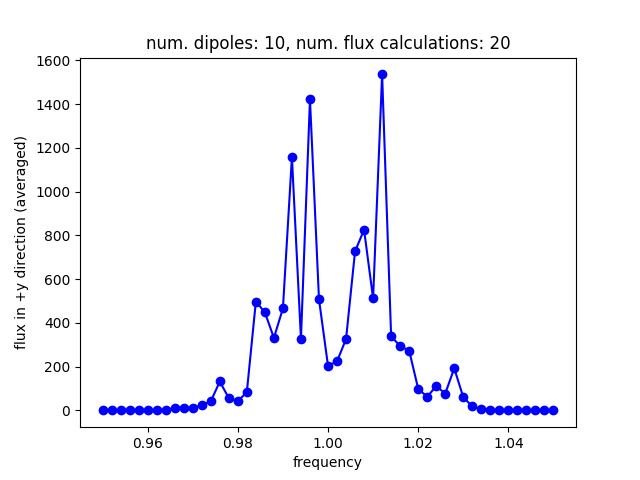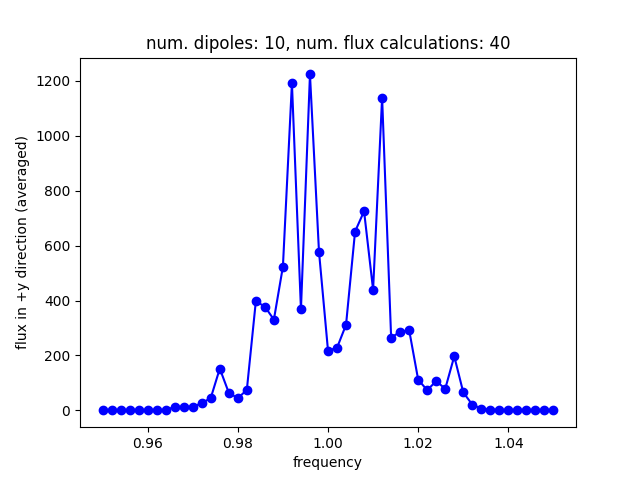点赞 评论 复制链接分享
• The easiest thing would be to nondimensionalize: compute the same thing for a structure with no surface-corrugation, and take the ratio.

Then, for approach (2), you don't need any fancy normalization — just use the same gaussian sources for all of the point-source cancellation, and the source spectrum will cancel when you normalize against the flat-surface emission.

点赞 评论 复制链接分享